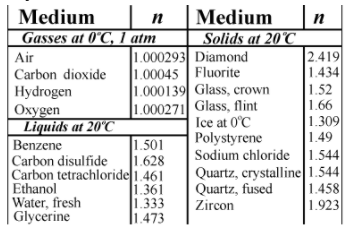# Problem: Suppose the speed of light in a particular medium is 2.012x108 m/s. Part A. Calculate the index of refraction for the medium. Part B. Based on the table identify the most likely substance.

###### FREE Expert Solution

Part A

Relationship between the speed of light and refractive index:

$\overline{){\mathbf{\eta }}{\mathbf{=}}\frac{\mathbf{c}}{\mathbf{v}}}$

80% (194 ratings)###### Problem Details

Suppose the speed of light in a particular medium is 2.012x108 m/s.

Part A. Calculate the index of refraction for the medium.

Part B. Based on the table identify the most likely substance.